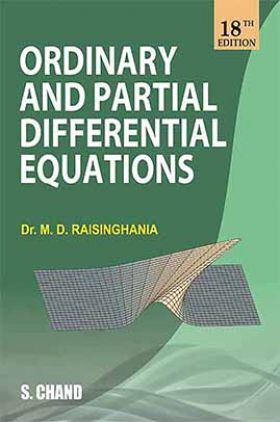# Ordinary and Partial Differential Equations

Sold ( 107 times )
165189 Views
MRP : ₹725.00
Price : ₹619.88
You will save : ₹105.13 after 15% Discount
Inclusive of all taxes

Save extra with 2 Offers

Get ₹ 50

Instant Cashback on the purchase of ₹ 400 or above

Product Specifications

 Publisher SChand Publications All M.Sc - Master of Science books by SChand Publications ISBN 9789385676161 Author: M D Raisinghania Number of Pages 1161 Available Available in all digital devicesOrdinary and Partial Differential Equations by M D Raisinghania

Book Summary:

This book has been designed for the use of honours and postgraduate students of various Indian universities. It will also be found useful by the students preparing for various competitive examinations.Do not start this book with an unreasonable fear. There are no mysteries in Mathematics. It is all simple and honest reasoning explained step by step which anybody can follow with a little effort and concentration. Often a student has difficulty in following a mathematical explanation only because the author skips steps which he assumes the students to be familiar with. If the student fails to recount the missing steps, he may be faced with a gap in the reasoning and the author’s conclusion may become mysterious to him.

Questions asked in recent papers of GATE and various university examinations have been inserted at appropriate places. This enriched inclusion of solved examples and variety of new exercises at the end of each article and chapter makes this book more useful to the reader.

Audience of the Book :
[For BA, B.Sc. and Honours (Mathematics and Physics), M.A., M.Sc. (Mathematics and Physics), B.E. Students of Various Universities and for I.A.S., P.C.S., A.M.I.E. GATE, C.S.I.R. U.G.C. NET and Various Competitive Examinations]

Key Features:

The following major changes have been made in the present edition:

• Almost all the chapters have been rewritten so that in the present form, the reader will not find any difficulty in understanding the subject matter.
• The matter of the previous edition has been re-organised so that now each topic gets its proper place in the book.
• More solved examples have been added so that the reader may gain confidence in the techniques of solving problems.
• References to the latest papers of various universities and I.A.S. examination have been made at proper places.
• Errors and omissions of the previous edition have been corrected

1. PART-I: ELEMENTARY DIFFERENTIAL EQUATIONS

• Differential Equations, their Formation and Solutions

• Equations of First Order and First Degree

• Trajectories

• Equations of the First Order but not of the First Degree Singular Solutions and Extraneous Loci

• Linear Differential Equations with Constant Coefficients

• Homogeneous Linear Equations or Cauchy-Euler Equations

• Method of Variation of Parameters

• Ordinary Simultaneous Differential Equations

• Exact Differential Equations and Equations of Special Forms

• Linear Differential Equations of Second Order

• Applications of Differential Equations

2. PART-II: ADVANCED ORDINARY DIFFERENTIAL EQUATIONS AND SPECIAL FUNCTIONS

• Picard's Iterative Method. Uniqueness and Existence Theorem

• Simultaneous Differential Equations of the form (dx)/P = (dy)/Q = (dz)/R

• Total (or Pfaffian) Differential Equations

• Beta and Gamma Functions

• Chebyshev Polynomials

• Fourier Series • Power Series

• Integration in Series

• Legendre Polynomials

• Legendre Functions of the Second Kind

• Bessel Functions • Hermite Polynomials

• Laguerre Polynomial

• Hypergeometric Function

• Orthogonal Set of Functions and Strum-Liouville Problem

3. PART-III: PARTIAL DIFFERENTIAL EQUATIONS

• Origin of Partial Differential Equations

• Linear Partial Differential Equations of Order One

• Non-Linear Partial Differential Equations of Order One

• Homogeneous Linear Partial Differential Equations with Constant Coefficients

• Non-Homogeneous Linear Partial Differential Equations with Constant Coefficients

• Partial Differential Equations Reducible to Equations with Constant Coefficients

• Partial Differential Equations of order two with Variables Coefficients

• Classification of Partial Differential Equations Reduction to Cononial or Normal Form. Riemann Method

• Monge's Method

• Transport Equation

• Cauchy Initial Value Problem for Linear First Order Partial Differential Equations